# IF Function in Google Sheets

The IF function in Google Sheets is used to make decisions with your data. It’s from the Logical family of functions in Google Sheets.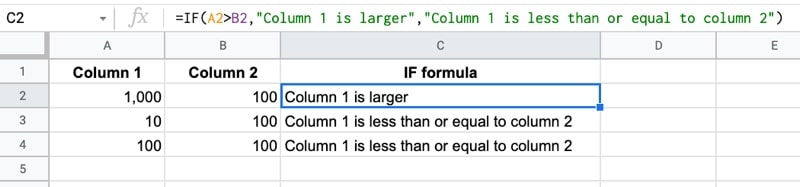In this tutorial, you’ll see how to use IF formulas in Google Sheets to make decisions with your data.

## What does the IF Function do?

At its heart, the IF function is a test that evaluates to a true or false value with a defined behavior if the outcome is true and a different behavior when the outcome is false.

Let’s see an example.

Suppose you have two columns of numbers in A and B and you want to compare each row to see which column has the larger number.

You would use the IF function in Google Sheets to do this!`=IF( A2 > B2 , "Column 1 is larger" , "Column 1 is less than or equal to column 2" )`

Inside the IF formula, the first expression `A2 > B2` checks whether the value in cell A2 is greater than the value in cell B2.

The outcome of this test is either a TRUE or a FALSE value.

The IF function requires a TRUE or FALSE value for this first argument.

Next up, you specify what you want to happen when the result is true. In this example the output of the function in the cell is “Column 1 is larger”

The final argument is the value you want to show if the result is false. In this example, that means the number in column A was smaller (or equal to) the value in column B. In this case, the output of the function is “Column 1 is less than or equal to column 2”.

## IF Function in Google Sheets: Syntax

` =IF(logical_expression, value_if_true, value_if_false)`

You might hear it referred to as an IF function, an IF formula or even an IF statement, but they all mean the same thing.

It takes 3 arguments:

`logical_expression`

An expression that gives a TRUE or FALSE answer, or a cell that contains a TRUE or FALSE value.

`value_if_true`

The value displayed by the IF function if the logical expression has the TRUE value.

`value_if_false`

The value displayed by the IF function if the logical expression has the FALSE value.

## IF function template

Feel free to make a copy: File > Make a copy…

If you can’t access the template, it might be because of your organization’s Google Workspace settings. If you click the link and open in an Incognito window you’ll be able to see it.

The IF function is also covered in the Day 2 lesson of my free Advanced Formulas 30 Day Challenge course.

## Calculations with the IF Function in Google Sheets

IF functions can be combined with other functions to perform calculations on values above a certain threshold for example.

When the logical expression is true, do this calculation, otherwise leave the value as it is.

Perhaps your business has a performance bonus structure that pays out a 20% bonus above a certain threshold of client revenue. Use an IF statement to determine if the threshold has been met and then put the calculation in the true field:

`=IF( A1 > 100000 , A1 * 0.2 , 0 )`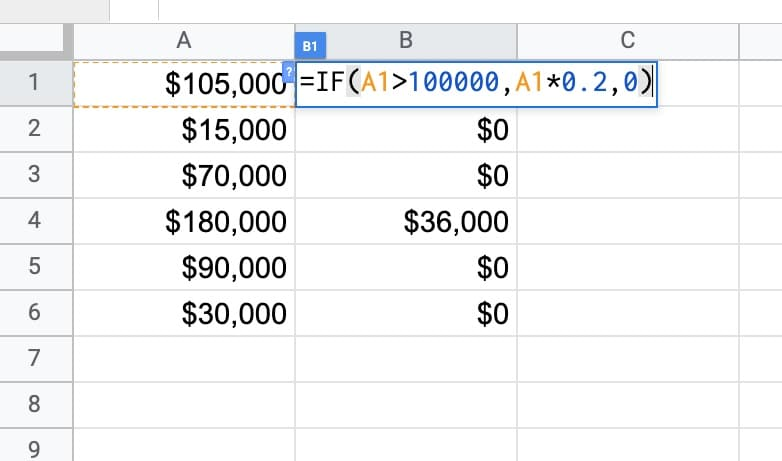## Using The IF Formula for Classification

Another example use case for the IF function is to classify items.

For example, you might want to segment your customers into long-term customers and new customers, based on whether they’ve been a client for 12 months or longer.

Suppose column A was a column containing the number of months a client has been with you, the following IF formula would classify them into long-term or new clients:

`=IF( A1 >= 12 , "Long-term client" , "New client" )`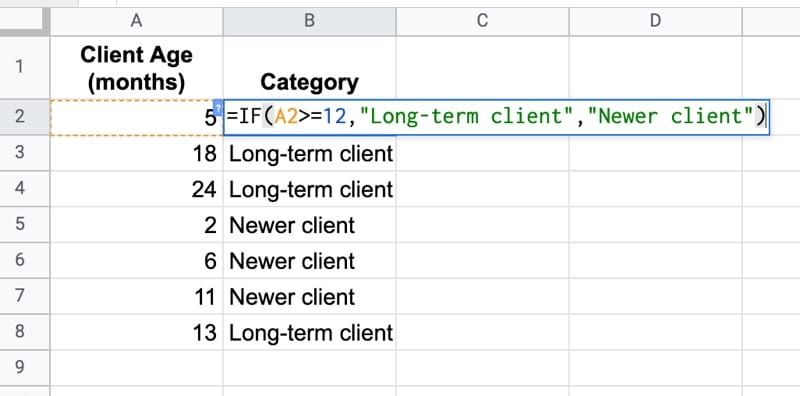This kind of segmentation is useful in lots of different ways.

It lets you compare the retention and churn metrics of the two groups. You can run different marketing campaigns to different segments of your data. Or, maybe you just want to send a “Thank you, you’re awesome!” card to your long-term clients.

## Nested IF Formula in Google Sheets

Sometimes one IF function alone isn’t enough.

Inside the TRUE or the FALSE arguments you can nest another IF function.

It looks complex but if you apply the onion method for working with formulas and work in layers, you’ll see it’s not difficult.

Working from the outside in, it checks if the value is greater than 50. If that is true, then the formula is done and it displays “Greater than 50”.

But if that’s false, it must mean the value is either less than 50 or equal to 50, so we use a second, nested IF to check for that:

`=IF(A2 > 50,"Greater than 50",IF(A2 < 50,"Less than 50","Equal to 50"))`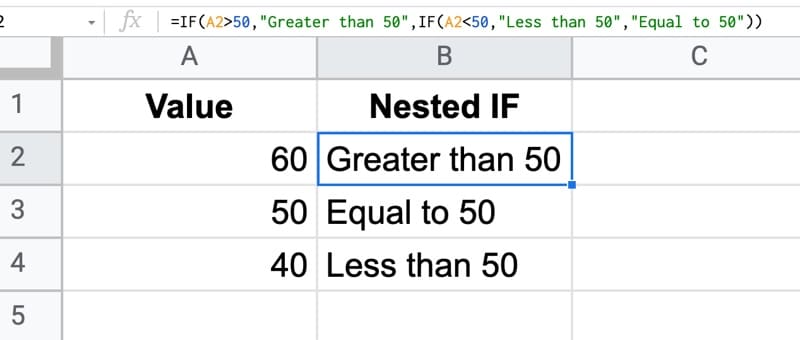If your nested IF formulas get too complicated, you might want to try the SWITCH function.

## IF Formulas and Checkboxes

Combining checkboxes with IF formulas is a powerful and versatile technique to use in your Sheets.

Checkboxes are actually just TRUE or FALSE values in disguise!

With a checkbox in cell A2, it can only ever have a TRUE or FALSE value. So it can be plugged into the IF function’s first argument directly!

`= IF( A2 , "It's checked!" , "It's not checked." )`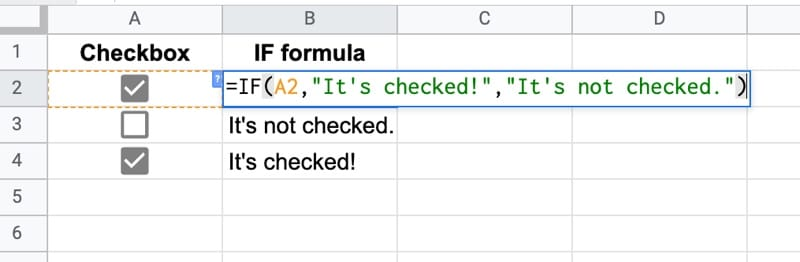This sort of logic is useful in many settings, for example a dashboard where you want to show/hide additional context for specific numbers or charts.

### Direct TRUE or FALSE Input

In the example above, you had a formula already in column A that contained a TRUE or FALSE value.

Since the first argument of the IF statement is looking for a TRUE of FALSE, you only need to reference that cell directly without testing for TRUE / FALSE.

Your IF formula would look like this:

`=IF( A1 , "Something true" , "Something false" )`

There is NO need to test for TRUE or FALSE again. So you should not see an IF function like this:

`=IF( A1 = TRUE , "Something true" , "Something false" )`

In fact, you should never see an “= TRUE” or “= FALSE” in an IF statement because they’re redundant.

## Replace numerical IF formulas with MIN or MAX functions

Suppose you have a column of values that you want to cap at a certain level, so that everything above a threshold value, e.g. 200, gets set to that value.

Most of us would approach this by writing an IF formula that checks whether the value is above 200 and then set it to 200 if TRUE, or the actual value if FALSE, like so: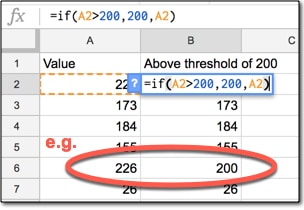However, you can replace the whole IF function with a much more succinct MIN function, which chooses the value 200 if the actual value is larger, since 200 is the minimum:

`=MIN( A2 , 200 )`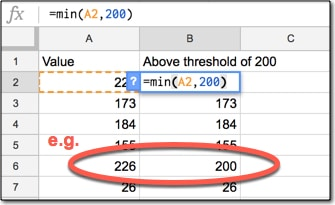Similarly, we can use the MAX function to replace IF statements when we’re looking at a threshold on the low side.

It’s good practice to write efficient formulas because it’s quicker and you’re less likely to make mistakes.

## 4 thoughts on “IF Function in Google Sheets”

1.Ahmad Saleem says:

Hi, I am struggling to perform IF on the “Date”. I have a field where there is a data and I want to use “IF” to update another field to highlight whether the date is “Greater” or “Equal” to 01 December 2021 and return “Yes” or “No”. I am unable to work out.

1.Nagesh Setty KS says:

Hope you can use this method
let the Column A2:A have the dates you want to compare with 1st Dec 2021, and Cell B1 has 1st Dec 2021
then the formula would be at the Cell B2 as below
=if(A2:A>=\$B\$1,”YES”,”NO”)

2.Andrew says:

Can you use cell references within the IF statement as one of the outputs? I’m using this for a particular sports stat calculation. I have something like =IF(T2>T1, “ARI”, “SEA”) but I want the form to automatically pick up the teams (ARI and SEA) from a data validation dropdown menu. I’ve tried different combinations of single quotes, double quotes, and the & sign but can’t quite figure it out. I know for a query string it’s ‘“&cell&”’ but that format won’t work for IF statements. There’s something I’m missing or don’t fully understand.

3.Russell says:

Hello Everyone is it possible to reference a cell that is a date when a check box is checked, If check box checked Cell K equals Cell B
Column B is invoice date ( the day invoices are received)
Column C is Paid at purchase ( Check Box)
Column K is the date of Column B if Column C is checked

=if(C4,”=B4″,” “)

I’m very new at this